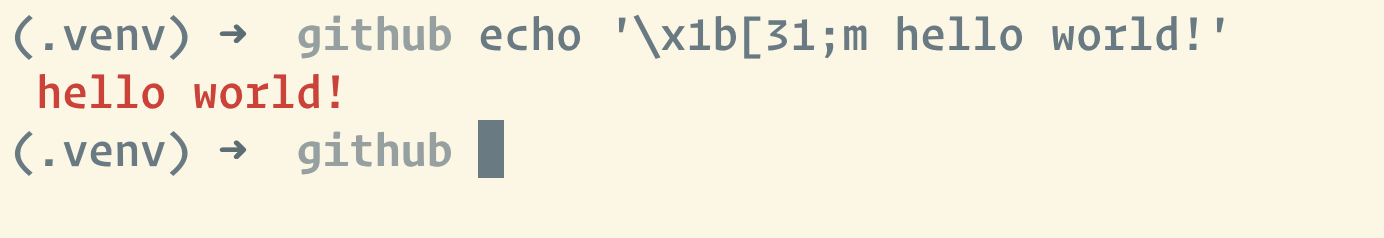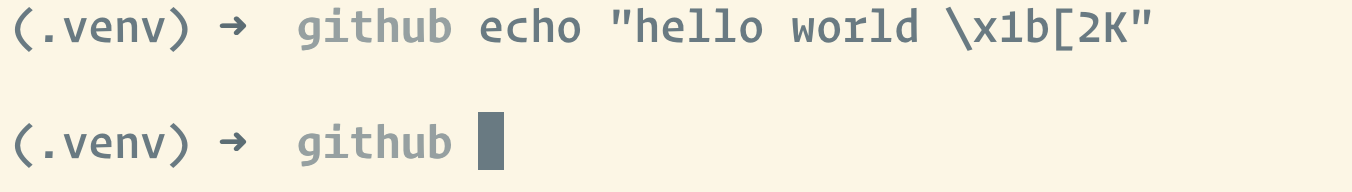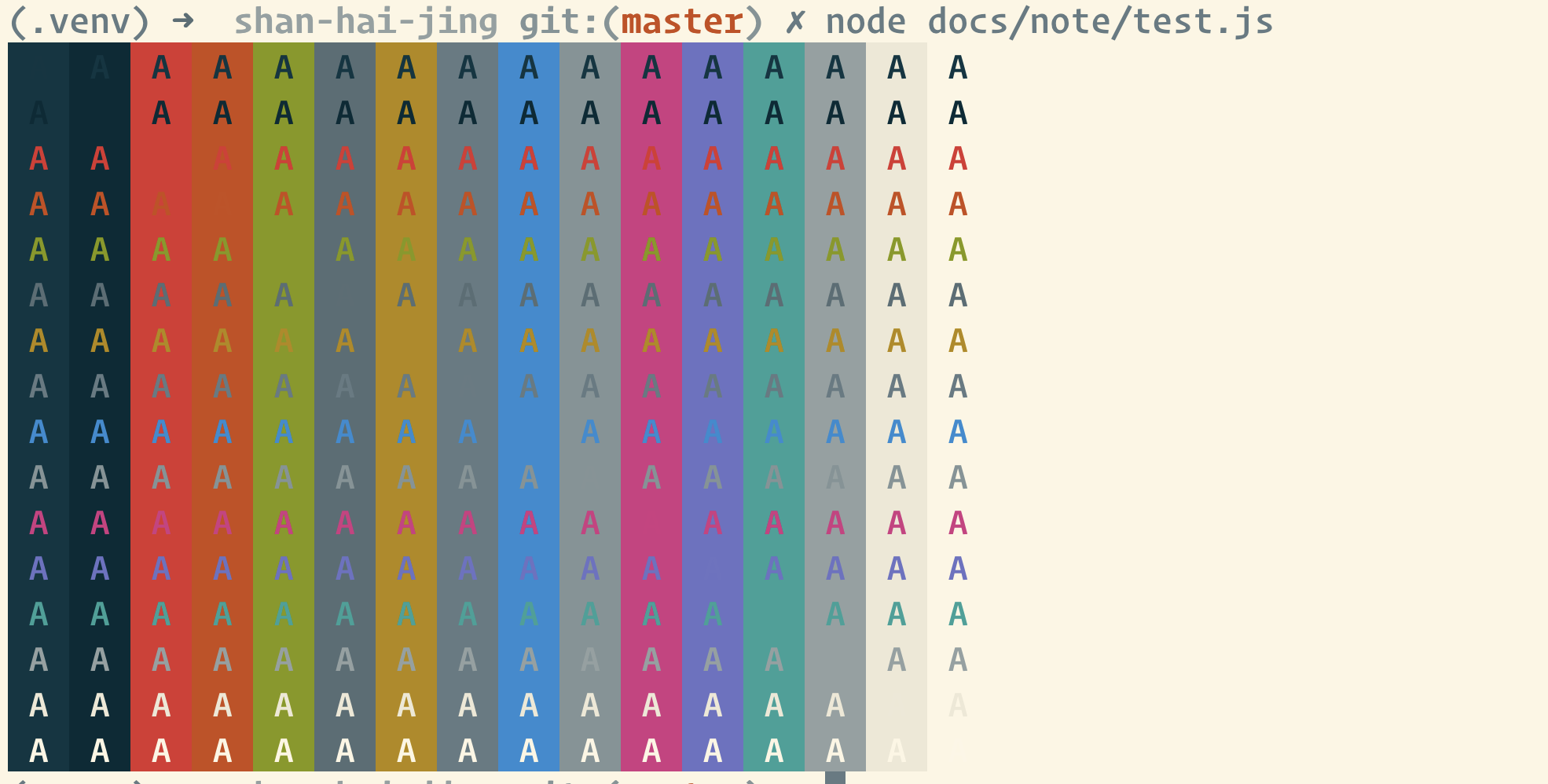feiker's blog

github

#### 任务分解

1. 传入一个进度数值 X，绘制 100%的进度容器和 X%的进度条部分部分 这个实现简单，只需要 X% 部分和(100-X)%部分的字符不同即可。

2. 每次进度变化，清除当前已绘制部分，绘制更新后的进度内容 在浏览器端实现这点很容易，清除绘制部分都由渲染进程处理，在命令行终端可以用 ANSI 转义序列控制字符绘制，具体可以参考 维基百科

#### ANSI

For Many Examples,

• 显示红字

``````echo '\x1b[31;m hello world!'
``````• 光标移动，清除整行

``````echo "hello world \x1b[2K"
``````• 来一手五彩斑斓的 A

``````const forecolor = [];
const backcolor = [];

for (let i = 30; i <= 37; i++) {
forecolor.push(i, i + 60);
backcolor.push(i + 10, i + 70);
}

for (let f of forecolor) {
let str = '';
for (let b of backcolor) {
str += `\x1b[\${f};\${b}m A `;
}
str += `\x1b[2k`;
console.log(str);
}
``````#### 简易进度条

``````const prefix = '[';
const postfix = ']';
const MAX_CHARS = 40;

function render(precent: number) {
const arrowNumber = ~~(MAX_CHARS * precent);
const bar = '>'.repeat(arrowNumber) + ' '.repeat(MAX_CHARS - arrowNumber);
const curProcessbar = `\x1b[1A\x1b[2K\${prefix}\${bar}\${postfix} \${Math.floor(
precent * 100
)}%`;
console.log(curProcessbar);
}

let cur = 0;
const timer = setInterval(() => {
if (cur >= 100) {
clearInterval(timer);
return;
}
cur++;
render(cur / 100);
}, 16);
````````````const MAX_CHARS = 40;

function render(precent: number) {
const arrowNumber = ~~(MAX_CHARS * precent);
const bar =
'\x1b[31m' +
'▓'.repeat(arrowNumber) +
'\x1b[34m' +
'░'.repeat(MAX_CHARS - arrowNumber);
const curProcessbar = `\x1b[1A\x1b[2K\${bar} \${Math.floor(precent * 100)}%`;
console.log(curProcessbar);
}

let cur = 0;
const timer = setInterval(() => {
if (cur >= 100) {
clearInterval(timer);
return;
}
cur++;
render(cur / 100);
}, 16);
``````### 总结

ANSI 转义序列（ANSI escape sequences）是一种带内信号的转义序列标准，用于控制视频文本终端上的光标位置、颜色和其他选项。在文本中嵌入确定的字节序列，大部分以 ESC 转义字符和"["字符开始，终端会把这些字节序列解释为相应的指令，而不是普通的字符编码。

github 地址

ANSI 转义序列